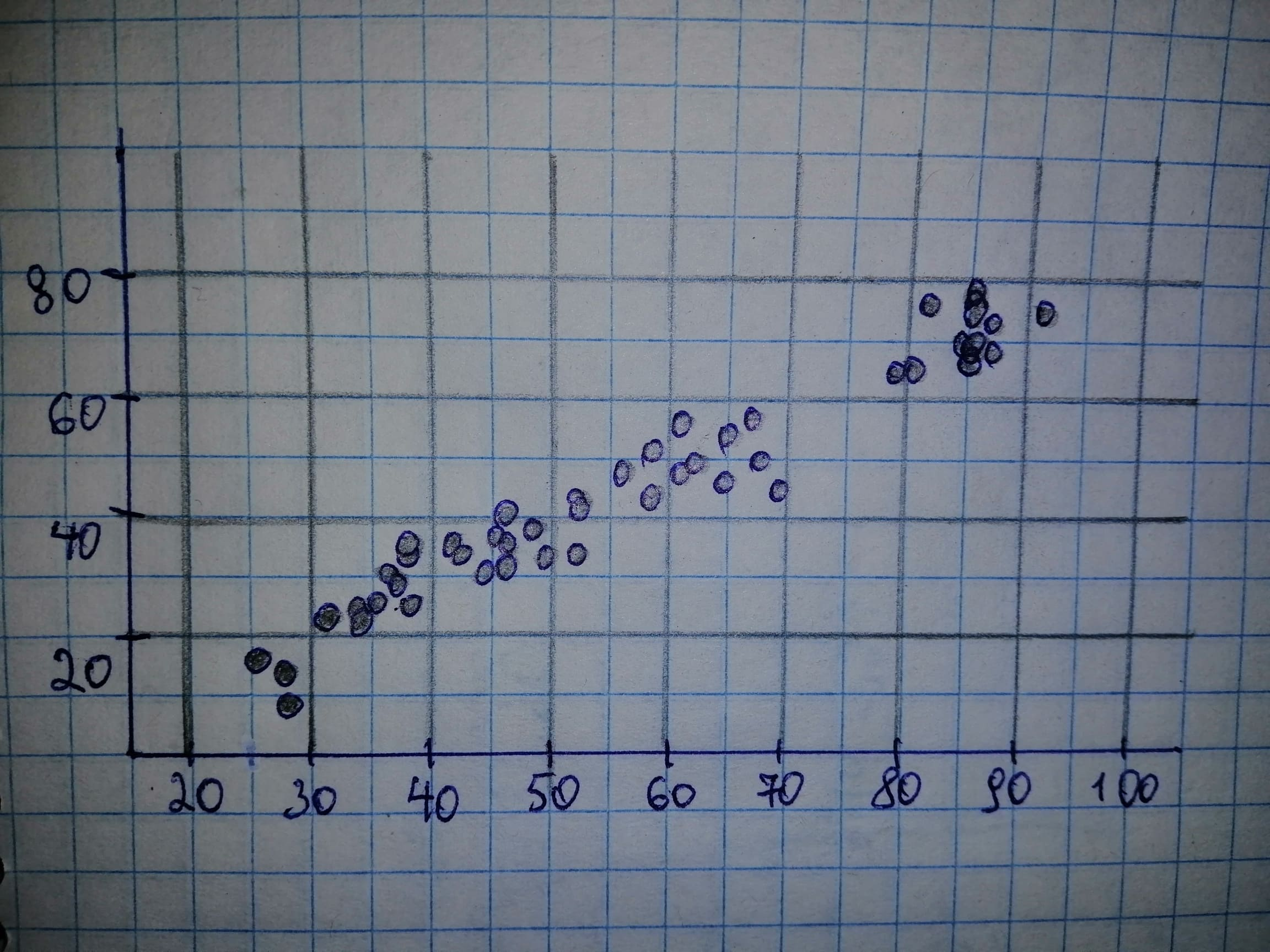Question# The National Oceanic and Atmospheric Administration publishes temperature information of cities around the world in Climates of the World.

Scatterplots
ANSWEREDThe National Oceanic and Atmospheric Administration publishes temperature information of cities around the world in Climates of the World. A random sample of 50 cities gave the data on average high and low temperatures in January shown.

a) Obtain a scatterplot for the data.

b) Decide whether finding a regression line for the data is reasonable. If so, then also do parts (c)-(f).

c) Determine and interpret the regression equation for the data.

d) Identify potential outliers and influential observations.

e) In case a potential outlier is present, remove it and discuss the effect.

f) In case a potential influential observation is present, remove it and discuss the effect.2021-03-03

Given: $$\displaystyle{n}=\ \text{Sample size}\ ={50}$$ a) High is on the horizontal axis and Low is on the vertical axis.b) It is reasonable to find a regression lien for the data if there is no strong curvature present in the scatterplot. We note that there is no strong curvature in the scatterplot of part (a) and thus it is reasonable to find a regression line for the data.

c) Let us first determine the necessary sums: $$\displaystyle\sum\ {x}_{{{i}}}={2843}$$
$$\displaystyle\sum\ {{x}_{{{i}}}^{{{2}}}}={181233}$$
$$\displaystyle\sum\ {y}_{{{i}}}={2228}$$
$$\displaystyle\sum\ {x}_{{{i}}}{y}_{{{i}}}={144636}$$ Next, we can determine $$\displaystyle{S}_{{\times}}$$ and $$\displaystyle{S}_{{{x}{y}}}$$
$$\displaystyle{S}_{{xx}}=\ \sum\ {{x}_{{{i}}}^{{{2}}}}\ -\ {\frac{{{\left(\sum\ {x}_{{{i}}}\right)}^{{{2}}}}}{{{n}}}}={181233}\ -\ {\frac{{{2843}^{{{2}}}}}{{{50}}}}={19580.02}$$
$$\displaystyle{S}_{{{x}{y}}}=\ \sum\ {x}_{{{i}}}{y}_{{{i}}}\ -\ {\frac{{{\left(\sum\ {x}_{{{i}}}\right)}{\left(\sum\ {y}_{{{i}}}\right)}}}{{{n}}}}={144636}\ -\ {\frac{{{2843}\ \cdot\ {2228}}}{{{50}}}}={17951.92}$$ The estimate b of the slope $$\displaystyle\beta$$ is the ratio of $$\displaystyle{S}_{{{x}{y}}}$$ and $$\displaystyle{S}_{{xx}}$$: $$\displaystyle{b}=\ {\frac{{{S}_{{{x}{y}}}}}{{{S}_{{xx}}}}}=\ {\frac{{{17951.92}}}{{{19580.02}}}}={0.9168}$$ The mean is the sum of all values divided by the number of values: $$\displaystyle\overline{{{x}}}=\ {\frac{{\sum\ {x}_{{{i}}}}}{{{n}}}}=\ {\frac{{{2843}}}{{{50}}}}={56.86}$$
$$\displaystyle\overline{{{y}}}=\ {\frac{{\sum\ {y}_{{{i}}}}}{{{n}}}}=\ {\frac{{{2228}}}{{{50}}}}={44.56}$$ The estimate a of the intercept $$\displaystyle\alpha$$ is the average of y decreased by the product of the estimate of the slope and the average of x. $$\displaystyle{a}=\ \overline{{{y}}}\ -\ {b}\ \overline{{{x}}}={44.56}\ -\ {0.9168}\ \cdot\ {56.86}=\ -{7.5720}$$ General least-squares equation: $$\displaystyle\hat{{{y}}}=\ \alpha\ +\ \beta\ {x}$$. Replace $$\displaystyle\alpha$$ by $$\displaystyle{a}=\ -{7.5720}$$ and $$\displaystyle\beta$$ by $$\displaystyle{b}={0.9168}$$ in the general least-squares equation: $$\displaystyle{y}={a}\ +\ {b}{x}=\ -{7.5720}\ +\ {0.9168}{x}$$

d) There appear to be no outliers, because no points in the graph deviate strongly from the general pattern in the other points. There appear to be no influential observatioons, because all data values lie near the regression line.

e) Not applicable, because we concluded that there are no potential outliers in part (d).

f) Not applicable, because we concluded that there are no potential outliers in part (d).